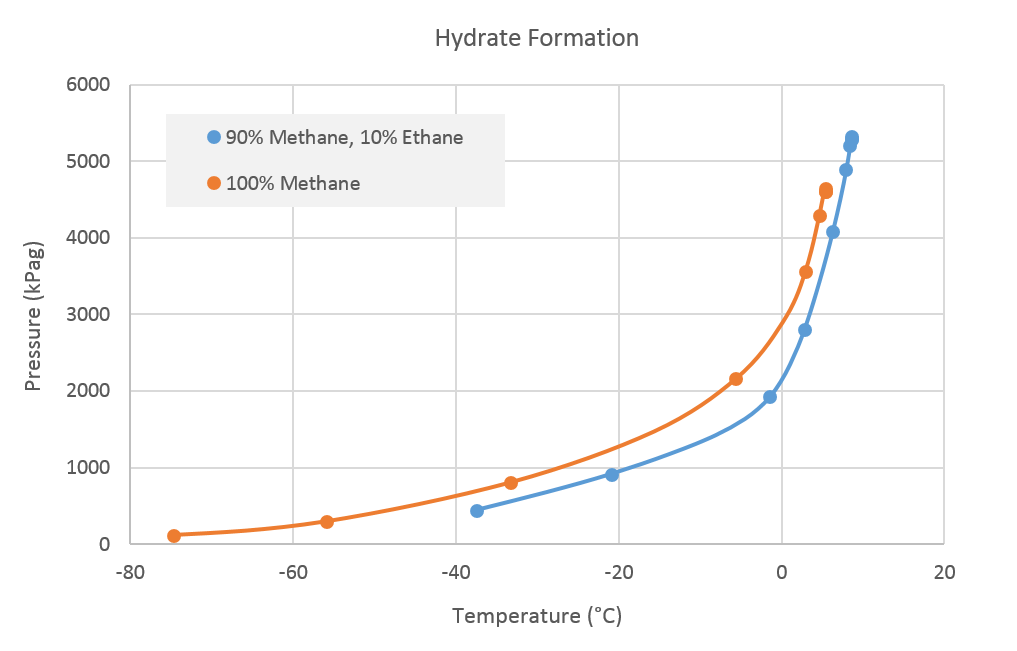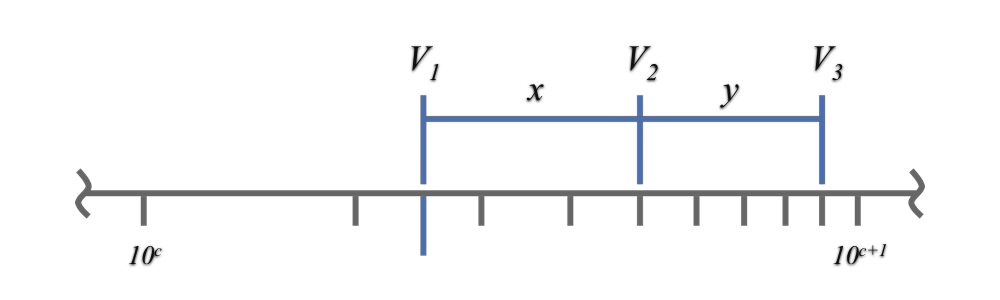f
Neutrium

# General-engineering

Index of Dimensionless Numbers

Dimensionless numbers play an important role in analysing fluid dynamics and heat and mass transfer problems. They provide a method by which complex phenomena can be characterised, often by way of a simple, single number comparison. This article provides a summary of dimensionless numbers and the formulae used to calculate them.

Dimensionless Numbers and Dimensional Analysis

Dimensionless numbers play an important role in analysing fluid dynamics and heat and mass transfer problems. They provide a method by which complex phenomena can be characterised, often by way of a simple, single number comparison. This article provides a brief overview of the derivation and use of dimensionless numbers.

Hydrate Formation in Gas SystemsHydrate formation represents a significant risk to process safety as it can result in the plugging of both pipes and instruments. Hydrates typically form in process where light hydrocarbons, water vapor and low temperatures or high pressures are present. This article describes the conditions under which hydrates form, how formation may be prevented and what can be done once hydrates have formed.

Baumé Scale

The Baumé scale was first developed in 1768 as a method of measuring the density of liquids. Today it is largely superseded, however it is still used in some industries as a measure of density or concentration. This article presents formula for the calculation of degrees Baumé for a liquid.

API Gravity

API gravity is measure of the density of liquid hydrocarbons relative to water. This article provides a reference to the API gravity calculation formula and some typical values of API gravity for common hydrocarbons.Reading values from a graph or plot with a logarithmic axis visually is difficult and will often result in inaccurate readings. This article presents formulae which may be used with measurements from a graph axis to obtain more accurate values.

Concentration Conversions

This article details the most common methods to quantify the concentration of a component in a mixture, and details how to convert between these concentrations. Concentration is commonly expressed in moles per volume, mass per volume, mass percentage, volume percentage, mole percentage and parts per million. Using density and/or molecular weight properties it is possible to convert between any of the above measures.

Ideal Gas Law

The ideal gas law is used to relate volume, pressure and temperature for gases. The ideal gas law is an approximation and is generally more accurate at mild temperatures and pressures. The properties of gases increasingly deviate from ideal as conditions become more extreme, i.e. low temperature or high pressure. In these cases a compressibility factor may be used to correct for non-ideal behaviour when using the ideal gas relationship.

Conversion of Standard Volumetric Flow Rates of Gas

Standard volumetric flow rates of a fluid are the equivalent of actual volumetric flow rates in the sense that they have an equal mass flow rate. This identity makes standard volumetric flow appropriate providing a common baseline for comparison of volumetric gas flow rate measurements at different conditions. This article outlines how to convert between standard and actual volumetric flow rates.